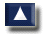# 4. ESTIMATES OF WATER STORAGE

## 4.0 Amount of water to be stored

You must first compare your pond water requirements - the water needed to initially fill your pond and to compensate for seepage and evaporation losses (see Section 2) - with the water available from your source (see Section 3).

If you find that your water source provides enough water to fill the pond in a reasonable period of time, to fill it when you want to fill it, and to compensate for water losses throughout the year, you will not need a reservoir.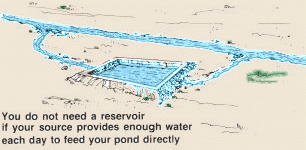If you find that your water source does not provide enough water to fill the pond and to compensate for water losses at certain times of the year, but there is enough water available over the year, you may decide to build a reservoir and store the water you will need. If you decide to build a reservoir, you will encounter one of two situations: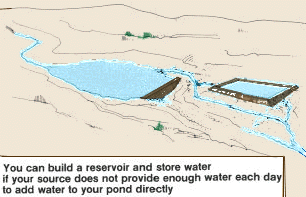### Your source provides a supply of water throughout the year

If your daily water flow is great enough throughout the year to compensate for water losses by seepage and evaporation, but not great enough to fill the pond in a reasonable period of time, and to fill it when you want to fill it, you will only need a reservoir with a volume equal to the pond volume, or even less, since the reservoir is being supplied constantly.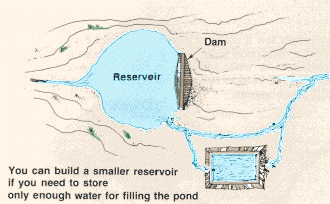Example

If your daily flow is not great enough throughout the year to fill the pond in a reasonable period of time when you want to fill it, and to compensate for water losses by seepage and evaporation, the reservoir will have to be large enough to provide for the total water requirements.

Example

### Your water source dries up completely at certain times of year

If your source does not supply water throughout the year, but dries up at certain times, you should plan to fill your pond during the year either from a reservoir, or directly from the source at a time when there is enough water available.

If you are able initially to fill your pond at a time when water is available, your reservoir need only contain enough water to compensate for water losses by seepage and evaporation during the dry season.

If you are unable initially to fill your pond at a time when there is water available, you may need to build a larger reservoir to store water over a longer period of time, and that will contain enough water to fill the pond and to compensate for water losses.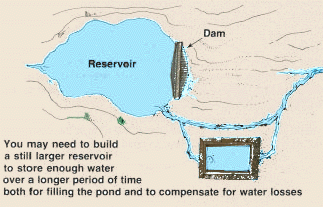### Water losses by seepage and evaporation from a reservoir

A reservoir is subject to water losses by seepage and evaporation just as a pond is. To offset water losses from a reservoir, plan the water volume of the reservoir 1.5 times greater than the water volume you require to satisfy total water requirements.

Example

## 4.1 Selecting a site for a reservoir

If you are going to build a reservoir, look for a site that will allow you to retain the greatest volume of water with the smallest dam possible. Dams require a great deal of work to build and to maintain, and the smaller the dam, the better.

Avoid a site in a valley, relatively open and wide at the downstream end. At such a site you will have to build a large dam.

### An unsatisfactory site for a reservoir

Wide contours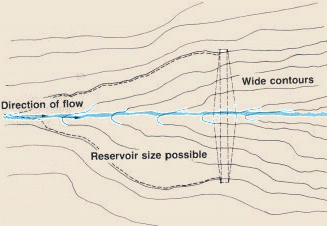The ideal site for a reservoir is in a wide valley which narrows suddenly, with steep sides at the downstream end. The narrower the valley end, the smaller the dam will have to be.

 Example A satisfactory site for a reservoir Close contours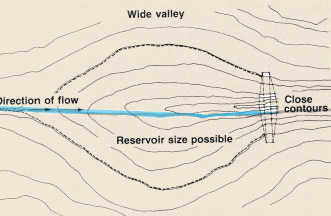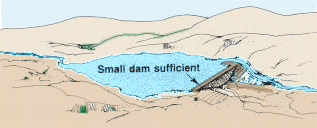Choose a site with good soil, which will hold water well. Avoid a sandy site. The place you choose should not have areas of sand that are too large to seal against water loss. If there are large sandy areas, it may be better to look for another site. It is good to clear the site of vegetation before building a reservoir. If you plan to do this, avoid choosing a site with too many large trees, which may be difficult to remove.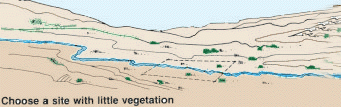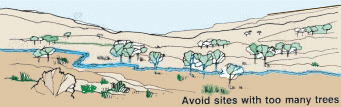You should choose a site where you can build a reservoir large enough to satisfy your total water requirements. It may be to your advantage to find more than one possible site and choose among them on the basis of: Your water requirements (see Section 2.3); How large a reservoir is possible at each site (see Section 4.2); The topography, soil and vegetation present at each site, as you have seen in this section.

 Example There are two possible sites on a stream at points A and B where conditions are suitable to build a dam for a reservoir. Both sites are equally suited as far as topography, soil and vegetation are concerned. Points A and B are near one another but note that point A is supplied by catchment basins 3, 4 and 5 while point B is supplied only by catchment basins 3 and 4. Therefore, more water can be collected at point A than at point B. But, before you decide which site to use, estimate your water requirements and pick the site with the smallest water capacity which best fits your requirements. Note: if the water flow of a stream increases greatly during the rainy season, it may be difficult to maintain a dam during this period. If the water level becomes too high the dam may be washed away. Example If the water flow increases greatly at points A and B during the rainy season, it will be safer to build a dam at point B where the water flow will be smaller.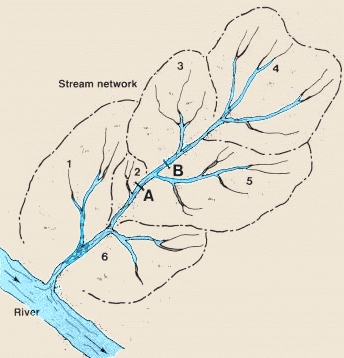## 4.2 Determining characteristics of a reservoir

When you have chosen a suitable site for a reservoir and have decided on the place to build the dam, you will have to determine the height of the dam you should build to store the volume of water required. To do this you will need to make one or more approximations to find the reservoir size that will give you the volume desired.

But first you will have to decide on the type of dam you will build. A dam may be built either without a spillway (when the stream water flow is relatively small and constant throughout the year) or with a spillway (when the stream flow is relatively large and/or when it greatly varies from one season to another). If conditions allow it, you should build a dam without a spillway, since it is easier to build. What is a spillway?

### Estimating the volume for a reservoir without a spillway

This is a relatively simple method that will give you a rough estimate of reservoir volume. If you plan to build a dam without a spillway, this rough approximation will be enough.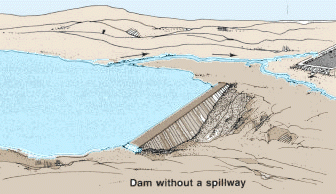### First approximation

Begin by assuming a maximum water depth at the dam of 1.5 m.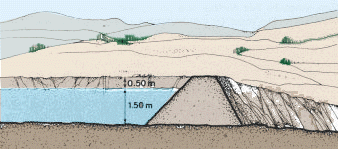Determine and mark the contour line at this assumed maximum water level using topographical measuring devices. The contour, when marked, will outline the extent of the planned reservoir.Now measure this contour line (in m) and calculate the approximate volume of water (in m3) that can be held in a reservoir of this size and maximum depth (1.5 m). To do this:

• Square the length of the contour line;
• Divide the result by 37.5 (conversion factor);
• Multiply by the assumed maximum water depth (in this case, 1.5 m) to find the approximate reservoir volume.

Example

 If this approximate water volume is between 10 and 20 percent greater than the water storage needed, you can build the dam and the reservoir based on the assumed depth of 1.5 m. Example You have estimated your water storage requirements as 2810 m3. Calculate a 10 percent and a 20 percent increase of this water storage requirement as (2840 m3 + 10%) and (2840 m3 + 20%) or 2840 + (2840 x 0.10) = 3124 m3 and 2840 + (2840 x 0.20)= 3408 m3. In the example above, you found the approximate reservoir volume to be 3340 m3, which is between 10 and 20 percent greater than the water storage requirement: 3124 m3 < 3340 m3 < 3408 m3. Therefore, the assumed maximum depth of 1.5 m can be accepted as the maximum water depth for this reservoir to satisfy water storage requirements.

If your first approximation is less than 10 percent or more than 20 percent of the water storage requirement, you will have to adjust the assumed maximum water depth and make another approximation.

If the approximate reservoir volume is much greater than the water storage requirement, reduce the assumed maximum water depth by 30 cm to 1.2 m and repeat the calculations, using this new assumed depth. If the calculated reservoir volume is still too great, reduce the assumed maximum water depth by another 30 cm to 0.90 m and repeat the process.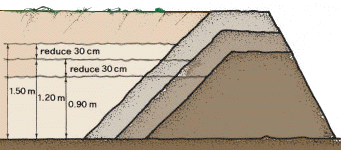If the calculated reservoir volume is much smaller than the water storage requirement, increase the assumed maximum depth by 30 cm to 1.8 m and repeat the calculations, using this new assumed maximum depth.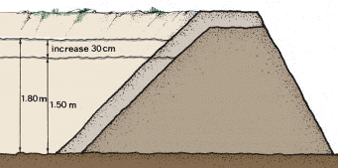### Estimating the volume for a reservoir with a spillway

If you decide to build a dam with a spillway, it will require more work and time than a dam without a spillway. Therefore, it is advisable to estimate the reservoir volume more accurately before you begin to build. This is a method that will allow you to calculate the volume of a planned reservoir with greater accuracy.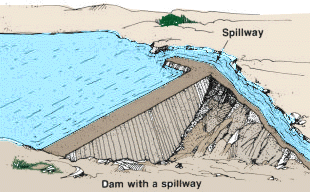To employ this method, you will need various topographical measuring devices for levelling - such as an A- frame, a straight edge and mason's level or other sighting device (that can also measure 90° angles) such as a cross staff, or a more sophisticated surveyor's level.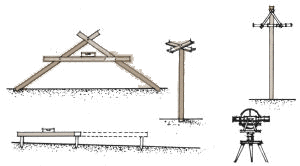### First calculation

Begin by assuming a maximum water depth of 1.5 m in the reservoir, when the water level reaches the crest of the spillway.Determine and mark the contour line at this assumed maximum water level, using a suitable levelling method. The contour, when marked, will outline the extent of the planned reservoir.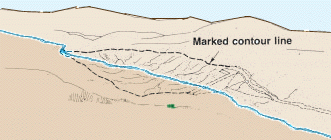Divide the planned reservoir area into 20 m x 20 m squares, using a suitable method for measuring 90° angles. In the centre of the area, you will have full squares but at the irregular edges of the reservoir you will have partial squares. Fix a wooden pole at the corner of each square within the reservoir area.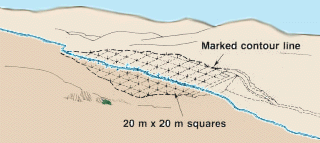Using a suitable leveling method, mark on the poles, at the corners of each square, the level that the water would reach if the maximum water depth of the reservoir were 1.5 m.

Now you are ready to calculate the water volume of each square. The sum of the volumes of all the squares will equal the total water storage volume of the reservoir.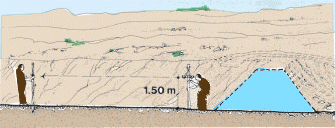### Calculate the volume of each full square

• Measure the water depth (in m) marked on the pole at each corner of the square;
• Add the four water depth figures and divide by 4 to find the average water depth in this square;
• Multiply the average water depth by 400 m2, which is the area of the 20 m x 20 m square, to find the total water volume of the square (in m3).Example

### Calculate the volume of each partial square

• Measure the water depth (in m) marked on the pole at the two corners of the square that are inside the planned reservoir area (the depth of the other two corners of the partial square is 0);
• Add the two water depth figures and divide by 4 to find the average water depth in this partial square;
• Measure the length of the two partial sides of the square (in m);
• Add these two length measurements and divide by 2 to find the average side length;
• Multiply this average side length by 20 m to find the area of the partial square (in m2);
• Multiply this area of the partial square by the average water depth to find the total water volume corresponding to the partial square (in m3).Example

After you have calculated the water volume for each full square and each partial square, add all these volumes to find the total water storage volume. This is the capacity of the planned reservoir if it has a maximum water depth of 1.5 m at the crest of the spillway.